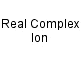[AgCl2]-
Dichlorosilver(I) ion
Silver(I) dichloro ionAg 1 Cl 2 charge = -1

Real ion.

Gram formula weight (molecular mass) = 178.77

The dichlorosilver(II) ion is linear.Dichlorosilver(I) ion
is defined with respect to the entities below:
The entities below are defined with respect to:
Dichlorosilver(I) ion
 Complex (AS/A2 generic)Complex anion Lewis base (generic)Real complex ion (generic)Type 23 Lewis acid/base complex (generic)VSEPR geometry = AX2 (generic)chemical compound molecule metal molecular science reaction mechanism ionic material acid base geometry reactivity synthesis science knowledge chemistry Lewis